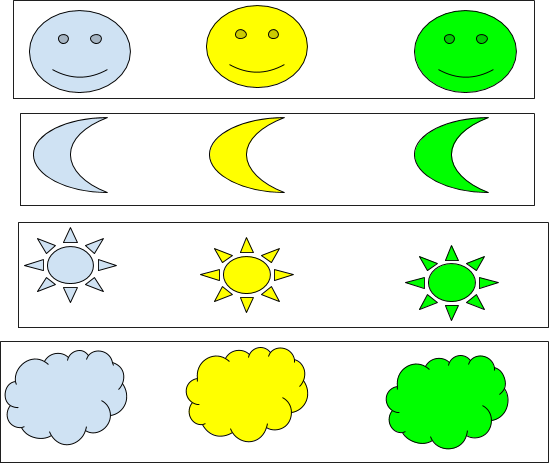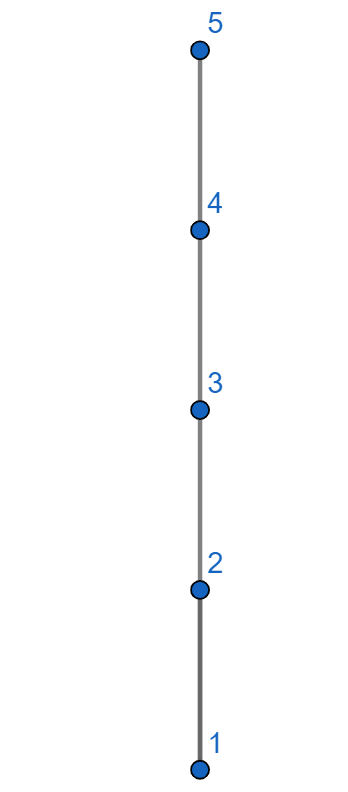# 2.2: Equivalence Relations, and Partial order

$$\newcommand{\vecs}{\overset { \rightharpoonup} {\mathbf{#1}} }$$ $$\newcommand{\vecd}{\overset{-\!-\!\rightharpoonup}{\vphantom{a}\smash {#1}}}$$$$\newcommand{\id}{\mathrm{id}}$$ $$\newcommand{\Span}{\mathrm{span}}$$ $$\newcommand{\kernel}{\mathrm{null}\,}$$ $$\newcommand{\range}{\mathrm{range}\,}$$ $$\newcommand{\RealPart}{\mathrm{Re}}$$ $$\newcommand{\ImaginaryPart}{\mathrm{Im}}$$ $$\newcommand{\Argument}{\mathrm{Arg}}$$ $$\newcommand{\norm}{\| #1 \|}$$ $$\newcommand{\inner}{\langle #1, #2 \rangle}$$ $$\newcommand{\Span}{\mathrm{span}}$$ $$\newcommand{\id}{\mathrm{id}}$$ $$\newcommand{\Span}{\mathrm{span}}$$ $$\newcommand{\kernel}{\mathrm{null}\,}$$ $$\newcommand{\range}{\mathrm{range}\,}$$ $$\newcommand{\RealPart}{\mathrm{Re}}$$ $$\newcommand{\ImaginaryPart}{\mathrm{Im}}$$ $$\newcommand{\Argument}{\mathrm{Arg}}$$ $$\newcommand{\norm}{\| #1 \|}$$ $$\newcommand{\inner}{\langle #1, #2 \rangle}$$ $$\newcommand{\Span}{\mathrm{span}}$$$$\newcommand{\AA}{\unicode[.8,0]{x212B}}$$

Definition: Equivalence Relation

A binary relation is an equivalence relation on a nonempty set $$S$$ if and only if the relation is reflexive(R), symmetric(S) and transitive(T).

Definition: Partial Order

A binary relation is a partial order if and only if the relation is reflexive(R), antisymmetric(A) and transitive(T).

Definition: Equivalence Class

Given an equivalence relation $$R$$ over a set $$S,$$ for any $$a \in S$$ the equivalence class of a is the set $$[a]_R =\{ b \in S \mid a R b \}$$, that is
$$[a]_R$$ is the set of all elements of S that are related to $$a$$.

Example $$\PageIndex{3}$$: Equivalence relation

Define a relation that two shapes are related iff they are the same color. Is this relation an equivalence relation?

Equivalence classes are:Example $$\PageIndex{4}$$:

Define a relation that two shapes are related iff they are similar. Is this relation an equivalence relation?

Equivalence classes are:##### Definition: Partition

Let $$A$$ be a nonempty set. A partition of $$A$$ is a set of nonempty pairwise disjoint sets whose union is A.

Note

For every equivalence relation over a nonempty set $$S$$, $$S$$ has a partition.

For the following examples, determine whether or not each of the following binary relationson the given setis reflexive, symmetric, antisymmetric, or transitive. If a relation has a certain property, prove this is so; otherwise, provide a counterexample to show that it does not. Ifis an equivalence relation, describe the equivalence classes of.

### Hasse Diagram

Consider the set $$S=\{1,2,3,4,5\}$$.  Then the relation $$\leq$$ is a partial order on $$S$$. Check!

Partial orders are often pictured using the Hasse diagram, named after mathematician Helmut Hasse (1898-1979).

Definition: Hasse Diagram

Let S be a nonempty set and let $$R$$ be a partial order relation on $$S$$. Then  Hasse diagram construction is as follows:

• there is a vertex (denoted by dots)  associated with every element of $$S$$.
• if $$a R b$$ , then the vertex $$b$$ is positioned higher than vertex  $$a$$.
• if $$a R b$$ and there is no $$c$$ such that $$a R c$$ and $$c R b$$, then a line is drawn from a to b.

This diagram is called the Hasse diagram.

##### Example $$\PageIndex{7}$$:

Hasse diagram for $$S=\{1,2,3,4,5\}$$ with the relation $$\leq$$.

Solution##### Example $$\PageIndex{8}$$:

Show that  $$\mathbb{Z}_+$$ with the relation $$|$$ is a partial order. Draw a Hasse diagram for  $$S=\{1,2,3,4,5,6\}$$ with the relation $$|$$.

SolutionThis page titled 2.2: Equivalence Relations, and Partial order is shared under a CC BY-NC-SA license and was authored, remixed, and/or curated by Pamini Thangarajah.